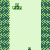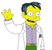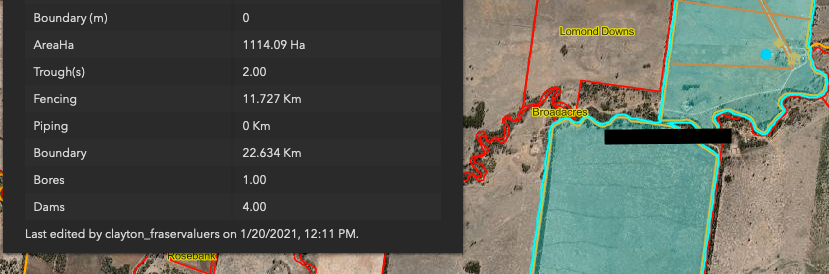# Calculated Field Automated in AGOL

441
3
03-16-2021 05:22 AM
Status: OpenNew Contributor II

Calculated Field Automated in AGOL.

In AGOL its only possible get calculations on a pop pup attribute expressions, but this funtionality its so more usable on the feature fields, a calculated field automaticly calculate when a create a new features would be more productive and open a new work possibilities.byWhat you're describing are essentially Attribute Rules, which, as you state, can be a huge productivity boost for various use cases.

AGOL does not have this capability that I'm aware of, and it remains one of the major selling points for moving to Portal and managing your own Enterprise Geodatabase.

Short of that, you could always have an automated script running every X minutes that looks at a service for new adds/edits and runs the desired calculation.You mean like in Excel? Where Column C = Column A + B for example. Or an acres column that auto calculates when geometry changes (it would have a formula converting sq ft to acres for example).  That would be nice.  Sure would...Hi,

I been using Acade functions within the popup design to display numbers of features within a polygon:Popup details

So there is scope to use calculations using Arcade functions to display in a popup, this one is used to calculate the lattitude and longitude details for a point:

• var x = text(geometry(\$feature).x)
var y = text(geometry(\$feature).y)
var originShift = 2.0 * PI * 6378137.0 / 2.0
var lon = (x / originShift) * 180.0;
var lat = (y / originShift) * 180.0;
• lat = 180.0 / PI * (2.0 * Atan( Exp( lat * PI / 180.0)) - PI / 2.0);

return text(Round(lat,6)+ "," + Round(lon,6))

Here are some resources on using Arcade fucntions:

CHeers

Nik